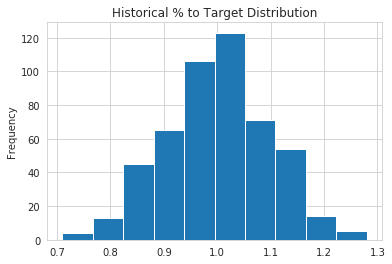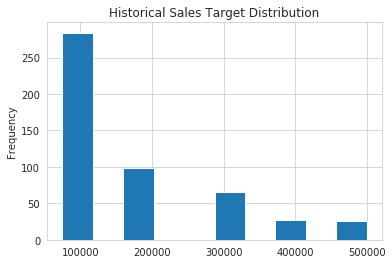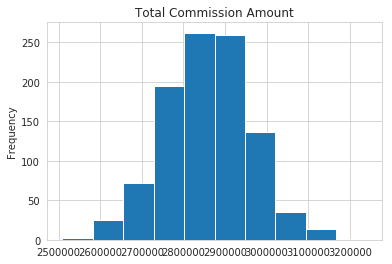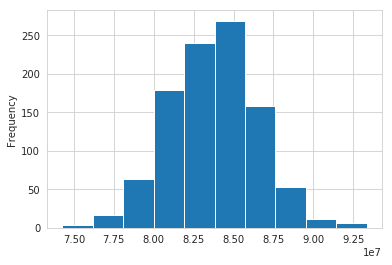### Monte Carlo Simulation with Python¶

Notebook to accompany article on Practical Business Python

In :
import pandas as pd
import numpy as np
import seaborn as sns

In :
sns.set_style('whitegrid')

In :
# Define the variables for the Percent to target based on historical results
avg = 1
std_dev = .1
num_reps = 500
num_simulations = 1000

In :
# Show an example of calculating the percent to target
pct_to_target = np.random.normal(avg, std_dev, num_reps).round(2)

In :
pct_to_target[0:10]

Out:
array([1.06, 1.27, 0.85, 0.91, 0.89, 1.18, 1.03, 0.83, 0.99, 0.89])
In :
# Another example for the sales target distribution
sales_target_values = [75_000, 100_000, 200_000, 300_000, 400_000, 500_000]
sales_target_prob = [.3, .3, .2, .1, .05, .05]
sales_target = np.random.choice(sales_target_values, num_reps, p=sales_target_prob)

In :
sales_target[0:10]

Out:
array([400000, 200000, 100000, 200000, 400000, 100000, 300000, 100000,
75000, 200000])
In :
# Show how to create the dataframe
df = pd.DataFrame(index=range(num_reps), data={'Pct_To_Target': pct_to_target,
'Sales_Target': sales_target})

Out:
Pct_To_Target Sales_Target
0 1.06 400000
1 1.27 200000
2 0.85 100000
3 0.91 200000
4 0.89 400000
In :
# Simple histogram to confirm distribution looks as expected
df['Pct_To_Target'].plot(kind='hist', title='Historical % to Target Distribution')

Out:
<matplotlib.axes._subplots.AxesSubplot at 0x7fd94422a2e8>In :
# Look at the sales target distribution
df['Sales_Target'].plot(kind='hist', title='Historical Sales Target Distribution')

Out:
<matplotlib.axes._subplots.AxesSubplot at 0x7fd9441fc668>In :
# Back into the actual sales amount
df['Sales'] = df['Pct_To_Target'] * df['Sales_Target']

In :
def calc_commission_rate(x):
""" Return the commission rate based on the table:
0-90% = 2%
91-99% = 3%
>= 100 = 4%
"""
if x <= .90:
return .02
if x <= .99:
return .03
else:
return .04

In :
df['Commission_Rate'] = df['Pct_To_Target'].apply(calc_commission_rate)

In :
df.head()

Out:
Pct_To_Target Sales_Target Sales Commission_Rate
0 1.06 400000 424000.0 0.04
1 1.27 200000 254000.0 0.04
2 0.85 100000 85000.0 0.02
3 0.91 200000 182000.0 0.03
4 0.89 400000 356000.0 0.02
In :
# Calculate the commissions
df['Commission_Amount'] = df['Commission_Rate'] * df['Sales']

In :
df.head()

Out:
Pct_To_Target Sales_Target Sales Commission_Rate Commission_Amount
0 1.06 400000 424000.0 0.04 16960.0
1 1.27 200000 254000.0 0.04 10160.0
2 0.85 100000 85000.0 0.02 1700.0
3 0.91 200000 182000.0 0.03 5460.0
4 0.89 400000 356000.0 0.02 7120.0
In :
print(df['Sales'].sum(), df['Commission_Amount'].sum(), df['Sales_Target'].sum())

87970500.0 3017002.5 87850000

In :
df.describe()

Out:
Pct_To_Target Sales_Target Sales Commission_Rate Commission_Amount
count 500.000000 500.000000 500.000000 500.000000 500.000000
mean 1.000760 175700.000000 175941.000000 0.033600 6034.005000
std 0.098028 121703.053645 123823.050417 0.007613 4745.301127
min 0.710000 75000.000000 58500.000000 0.020000 1170.000000
25% 0.930000 75000.000000 82500.000000 0.030000 2932.500000
50% 1.005000 100000.000000 108000.000000 0.040000 4180.000000
75% 1.070000 200000.000000 228000.000000 0.040000 8415.000000
max 1.280000 500000.000000 620000.000000 0.040000 24800.000000

### Show Full Example of the simulation¶

In :
# Define a list to keep all the results from each simulation that we want to analyze
all_stats = []

# Loop through many simulations
for i in range(num_simulations):

# Choose random inputs for the sales targets and percent to target
sales_target = np.random.choice(sales_target_values, num_reps, p=sales_target_prob)
pct_to_target = np.random.normal(avg, std_dev, num_reps).round(2)

# Build the dataframe based on the inputs and number of reps
df = pd.DataFrame(index=range(num_reps), data={'Pct_To_Target': pct_to_target,
'Sales_Target': sales_target})

# Back into the sales number using the percent to target rate
df['Sales'] = df['Pct_To_Target'] * df['Sales_Target']

# Determine the commissions rate and calculate it
df['Commission_Rate'] = df['Pct_To_Target'].apply(calc_commission_rate)
df['Commission_Amount'] = df['Commission_Rate'] * df['Sales']

# We want to track sales,commission amounts and sales targets over all the simulations
all_stats.append([df['Sales'].sum().round(0),
df['Commission_Amount'].sum().round(0),
df['Sales_Target'].sum().round(0)])

In :
results_df = pd.DataFrame.from_records(all_stats, columns=['Sales', 'Commission_Amount', 'Sales_Target'])

In :
results_df.describe().round(0).style.format('{:,}')

Out:
Sales Commission_Amount Sales_Target
count 1,000.0 1,000.0 1,000.0
mean 83,709,161.0 2,858,654.0 83,702,875.0
std 2,757,247.0 102,738.0 2,731,060.0
min 74,279,750.0 2,509,105.0 74,725,000.0
25% 81,792,875.0 2,790,037.0 81,800,000.0
50% 83,796,250.0 2,858,382.0 83,625,000.0
75% 85,533,000.0 2,925,028.0 85,600,000.0
max 93,342,750.0 3,240,805.0 92,800,000.0
In :
results_df['Commission_Amount'].plot(kind='hist', title="Total Commission Amount")

Out:
<matplotlib.axes._subplots.AxesSubplot at 0x7fd943b3f940>In :
results_df['Sales'].plot(kind='hist')

Out:
<matplotlib.axes._subplots.AxesSubplot at 0x7fd943b11630>